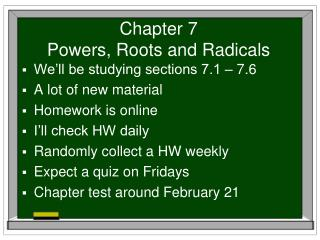DownloadDownload PresentationChapter 7 Powers, Roots and Radicals

# Chapter 7 Powers, Roots and Radicals

Download Presentation## Chapter 7 Powers, Roots and Radicals

- - - - - - - - - - - - - - - - - - - - - - - - - - - E N D - - - - - - - - - - - - - - - - - - - - - - - - - - -
##### Presentation Transcript

1. Chapter 7Powers, Roots and Radicals • We’ll be studying sections 7.1 – 7.6 • A lot of new material • Homework is online • I’ll check HW daily • Randomly collect a HW weekly • Expect a quiz on Fridays • Chapter test around February 21

2. nth Roots and Rational Exponents Section 7.1 p401

3. What we’ll talk about today • What is an nth root? • Radical and exponent notation • Real number roots • Rational exponents • Roots and your calculator • Solving an equation using nth roots

4. nth roots • Recall the definition of a square root • A number r is a square root of a number s if r 2 = s. • This means that r x r = s

6. Other roots • Extending the definition of square roots, if bn= a, then b is the nth root of a. • An nth root can be written as n√a, where n is the index of the radical.

7. Real nth roots

8. Rational Exponents • If √a = ak, then what is k? From the definition of square root: √a √a = a akxak=a1, so ak+k = a1 Since the bases are the same 2k = 1, k = ½ What does this mean? The rational exponent of ½ is the same as the square root.

9. Rational exponents Let a1/n be an nth root of a and let m be a positive integer. • am/n = (a1/n)m = (n√a)m

10. Examples of equivalent expressions

11. Write as equivalent expressions.

12. Using a calculator • Option 1: Use the rational exponent notation. Pay attention to parentheses and the order of operations! 102/5 would be entered as 10^(2/5) ≈2.512

13. Evaluate to the nearest hundredth. ≈ 43.22 ≈ 0.12

14. Evaluate to the nearest hundredth. ≈ 43.22 ≈ 0.12

15. Using a calculator • Option 2: Use the radical notation. Use parentheses! would be entered as 6 (index) MATH 5:x√ (530)^7

16. Without a calculator, find the indicated nth root of a. Use prime factorization if you are stuck. • n = 5, a = -32 • n = 4, a = -81 no real solution • n = 4, a = 16

17. Solving equations using nth roots • How do you think we’ll solve these equations: